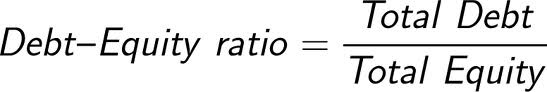# What Is The Debt To Equity Ratio?A measure of a company’s financial leverage. Debt/equity ratio is equal to long-term debt divided by common shareholders’ equity. Typically the data from the prior fiscal year is used in the calculation. Investing in a company with a higher debt/equity ratio may be riskier, especially in times of rising interest rates, due to the additional interest that has to be paid out for the debt.

For example, if a company has long-term debt of \$3,000 and shareholder’s equity of \$12,000, then the debt/equity ratio would be 3000 divided by 12000 = 0.25. It is important to realize that if the ratio is greater than 1, the majority of assets are financed through debt. If it is smaller than 1, assets are primarily financed through equity.